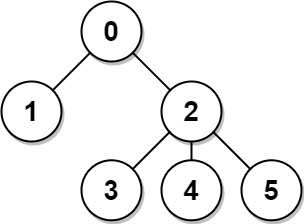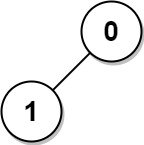834. Sum of Distances in Tree
Hard
4.5K
104

There is an undirected connected tree with `n` nodes labeled from `0` to `n - 1` and `n - 1` edges.

You are given the integer `n` and the array `edges` where `edges[i] = [ai, bi]` indicates that there is an edge between nodes `ai` and `bi` in the tree.

Return an array `answer` of length `n` where `answer[i]` is the sum of the distances between the `ith` node in the tree and all other nodes.

Example 1:```Input: n = 6, edges = [[0,1],[0,2],[2,3],[2,4],[2,5]]
Output: [8,12,6,10,10,10]
Explanation: The tree is shown above.
We can see that dist(0,1) + dist(0,2) + dist(0,3) + dist(0,4) + dist(0,5)
equals 1 + 1 + 2 + 2 + 2 = 8.
Hence, answer = 8, and so on.
```

Example 2:```Input: n = 1, edges = []
Output: 
```

Example 3:```Input: n = 2, edges = [[1,0]]
Output: [1,1]
```

Constraints:

• `1 <= n <= 3 * 104`
• `edges.length == n - 1`
• `edges[i].length == 2`
• `0 <= ai, bi < n`
• `ai != bi`
• The given input represents a valid tree.
Accepted
80.8K
Submissions
136.7K
Acceptance Rate
59.1%

Seen this question in a real interview before?
1/4
Yes
No

Discussion (0)

Related Topics